Courses

# IIFT Data Interpretation MCQ Quiz - 1

## 18 Questions MCQ Test IIFT Mock Test Series | IIFT Data Interpretation MCQ Quiz - 1

Description
This mock test of IIFT Data Interpretation MCQ Quiz - 1 for CAT helps you for every CAT entrance exam. This contains 18 Multiple Choice Questions for CAT IIFT Data Interpretation MCQ Quiz - 1 (mcq) to study with solutions a complete question bank. The solved questions answers in this IIFT Data Interpretation MCQ Quiz - 1 quiz give you a good mix of easy questions and tough questions. CAT students definitely take this IIFT Data Interpretation MCQ Quiz - 1 exercise for a better result in the exam. You can find other IIFT Data Interpretation MCQ Quiz - 1 extra questions, long questions & short questions for CAT on EduRev as well by searching above.
QUESTION: 1

### Group Question Answer the following question based on the information given below. The two pie-charts below provide information about the background-wise batch distribution of a B-school class in 2011 and 2012. The total number of students from the Arts background increased by 25% in 2012 as compared to 2011. (Assume that the total number of students to be 300 in the year 2011)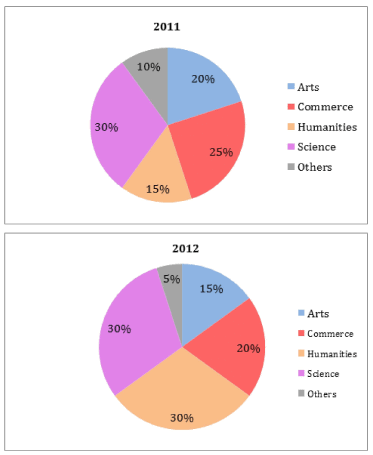Q. How many more students were there from the Humanities background in 2012 as compared to 2011 ?

Solution:

The total number of students from the Arts background increased by 25% in 2012 as compared to 2011. Number of students from the Arts background in 2011 = 20% of 300 = 60.
Therefore, number of students from the Arts background in 2012 = 1.25 x 60 = 75 This forms 15% of the total batch size in 2012.
So, total batch size in 2012 = 75/0.15 = 500. For 300 and 500 stuents in 2011 and 2012, the number of students from each background were: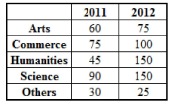Thus, there were 150 - 45 = 105 more students in the humanities background in 2012 as compared to 2011.
Hence, option 2.

QUESTION: 2

### The two pie-charts below provide information about the background-wise batch distribution of a B-school class in 2011 and 2012. The total number of students from the Arts background increased by 25% in 2012 as compared to 2011. (Assume that the total number of students to be 300 in the year 2011)Q. What was the percentage increase in the number of students from the Science background in 2012 as compared to 2011 ?

Solution:

Irrespective of the number of total students in 2011, the percentage change in the number of students from a particular background will remain the same.
Therefore, consider the solution to the previous question and assume the total number of students in 2011 to be 300.

So, the number of students from a Science background in 2011 and 2012 is 90 and 150 respectively.
Hence,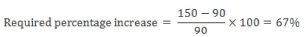Hence, option 4.

QUESTION: 3

### Group Question Answer the following question based on the information given below. The bar graph below shows the average electricity consumption in a house in all alternate years from 2000 to 2008. The payable amount for a particular year comprises the billed amount and 12.36% service tax. The service tax is calculated on the billed amount. The billed amount is calculated solely on the number of units consumed, and the units are billed as per various slabs (as shown in the table). For instance, if a household has consumed 420 units in a year, the first 100 units are charged at Rs. 2.23/unit, the next 300 emits are charged at Rs. 4.78/unit and the final 20 emits are charged at Rs. 6.78/unit.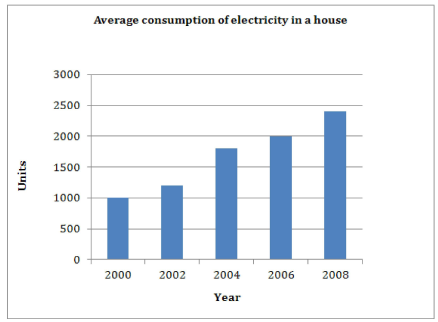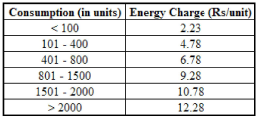Q. What is the payable amount for this household in 2006?

Solution:

Consumption of the household in 2006 = 2000 units

Therefore Billed amount = (100 x 2.23) + (300 x 4.78) + (400 x 6.78) + (700 x 9.28) + (500 x 10.78) = 223 + 1434 + 2712 + 6496 + 5390
= Rs. 16,255.

Payable amount = 16255 + (12.36% of 16255) = 1.1236 x 16255 ~ Rs. 18,264.

Hence, option 2.

QUESTION: 4

The bar graph below shows the average electricity consumption in a house in all alternate years from 2000 to 2008. The payable amount for a particular year comprises the billed amount and 12.36% service tax. The service tax is calculated on the billed amount. The billed amount is calculated solely on the number of units consumed, and the units are billed as per various slabs (as shown in the table). For instance, if a household has consumed 420 units in a year, the first 100 units are charged at Rs. 2.23/unit, the next 300 emits are charged at Rs. 4.78/unit and the final 20 emits are charged at Rs. 6.78/unit.Q. By what amount does the payable amount for 2004 exceed the payable amount for 2002?

Solution:

The number of units consumed in 2002 and 2004 is 1200 and 1800 respectively.
In 2004, the billed amount on the first 1200 units is the same as the billed amount for 2002.
So, the payable amount for the first 1200 units in 2004 is the same as the entire payable amount for 2002.
Thus, the required difference is entirely due to the additional 600 units consumed in 2004 and the service tax calculated on these 600 units. 300 of these 600 units fall in the 801-1500 slab and the remaining fall in the 1501-2000 slab.
Payable amount for the additional = 1.1236 x [(300 x 9.28) + (300 x 10.78)] = 1.1236 x (2784+ 3234) = Rs. 6761.82
Hence, option 3.

QUESTION: 5

The bar graph below shows the average electricity consumption in a house in all alternate years from 2000 to 2008. The payable amount for a particular year comprises the billed amount and 12.36% service tax. The service tax is calculated on the billed amount. The billed amount is calculated solely on the number of units consumed, and the units are billed as per various slabs (as shown in the table). For instance, if a household has consumed 420 units in a year, the first 100 units are charged at Rs. 2.23/unit, the next 300 emits are charged at Rs. 4.78/unit and the final 20 emits are charged at Rs. 6.78/unit.Q. How much service tax has the household paid in all over the given period?

Solution:

Since the service tax rate remains constant over the given period, the service tax can be calculated directly on the total billed amount instead of calculating the service tax for each billed amount.
Consider the solution to the first question. The billed amount for 2006 is Rs. 16,255 The billed amount for the other years is:

2000: (100 x 2.23) + (300 x 4.78) + (400 x 6.78) + (200 x 9.28) = 223 + 1434 + 2712 + 1856 = Rs. 6,225.

2002: (100 x 2.23) + (300 x 4.78) + (400 x 6.78) + (400 x 9.28) = 223 + 1434 + 2712 + 3712 = Rs. 8,081

2004: (100 x 2.23) + (300 x 4.78) + (400 x 6.78) + (700 x 9.28) + (300 x 10.78) = 223 + 1434 + 2712 + 6496 + 3234 = Rs. 14,099.

2008: (100 x 2.23) + (300 x 4.78) + (400 x 6.78) + (700 x 9.28) + (500 x 10.78) + (400 x 12.28) = 223 + 1434 + 2712 + 6496 + 5390 + 4912 = Rs. 21,167.

Total billed amount = 6225 + 8081 + 14099 + 16255 + 21167 = Rs. 65,827.

Total service tax = 12.36% of 65827 ~ Rs. 8,136 Hence, option 3.

QUESTION: 6

Group Question

Answer the following question based on the information given below.

The table below shows the number of male and female voters in eight constituencies of a state from 2012 to 2014.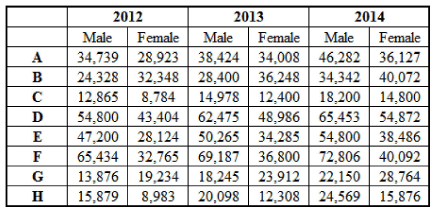Q. In how many constituencies is the compound annual growth rate (CAGR) of male voters less than that of the female voters?

Solution:

Because, the period is only 2 years, calculate the CAGR for male and female voters using the formula: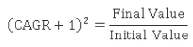Now, in a particular constituency, if the ratio on the RHS is higher for males, the males will have a higher CAGR in that constituency.
Hence, calculate only the ratio on the RHS, as shown below.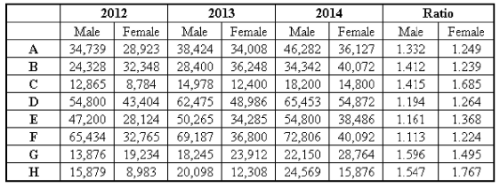Thus, the CAGR for males is less than that for females in five constituencies i.e. C, D, E, F and H.
Hence, option 3.

QUESTION: 7

The table below shows the number of male and female voters in eight constituencies of a state from 2012 to 2014.Q. Which constituency witnessed the highest percentage growth in the total number of voters, over the given period?

Solution:

Calculate the total number of voters in 2012 and 2014, and their corresponding percentage growth as shown below: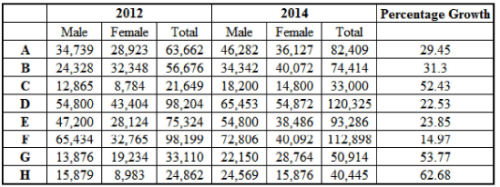Thus, the highest percentage growth is in constituency H. Hence, option 4.

QUESTION: 8

The table below shows the number of male and female voters in eight constituencies of a state from 2012 to 2014.Q. In which constituency is the CAGR of total voters the least over the given period?

Solution:

Consider the solution to the earlier questions.Now, the final value and initial value of total voters has been found earlier, as shown below.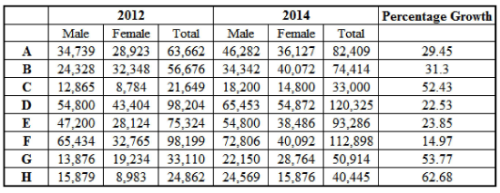As explained earlier, the constituency with the least ratio of final to initial voters will have the least CAGR.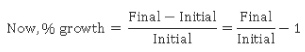Thus, the least constituency with the least percentage growth will have the least ratio, and hence, the least CAGR.
This constituency is F. Hence, option 1.

QUESTION: 9

Group Question

Answer the following question based on the information given below.

Year-wise break-up of number of educational institutions in India: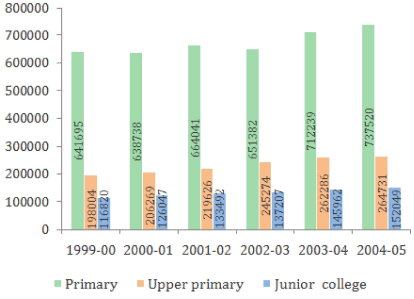Q. Which of the following year has seen the maximum percentage  growth of Upper primary schools over the previous year?

Solution:

Percentage growth of Upper primary schools: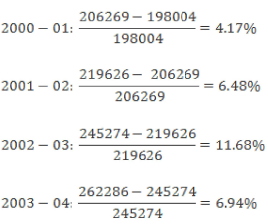The year 2002-03 has seen the maximum growth. Hence, option 3.

QUESTION: 10

Year-wise break-up of number of educational institutions in India:Q. Which of the following statements is definitely true?

Solution:

Option (1):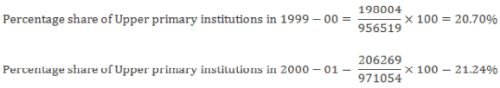Increase = 21.24 - 20.70 = 0.54%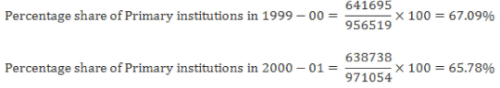Decrease = 67.09 - 65.78 = 1.31% Since the increase in percentage share of Upper primary institutions in 2000-01 is less than the decrease in percentage share of Primary institutions in the same period, the statement is false.

Option (2):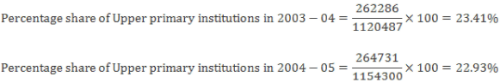Decrease = 23.41 - 22.93 = 0.48%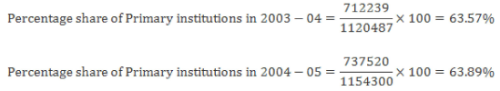Increase = 63.89 - 63.57 = 0.32% Since the decrease in percentage share of Upper primary institutions in 2004-05 is more than the increase in percentage share of Primary institutions in the same period, the statement is false.
Option (3):

From option (1), we have already seen that the year 2000-01 has also seen a decline in the percentage share of primary institutions, so the statement is false.
Option (4):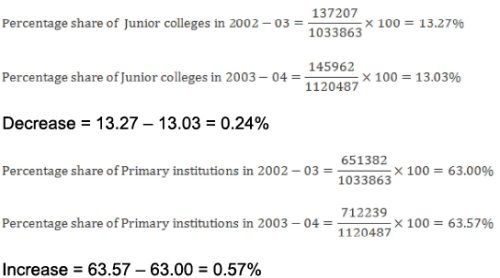Since the decrease in percentage share of Junior colleges in total in the year 2003-04 over the previous years is less than the increase in percentage share of primary institutions in the same period, the statement is true.
Hence, option 4.

QUESTION: 11

Year-wise break-up of number of educational institutions in India:Q. Which of the following statement(s) is/are definitely true?

Solution:

Option (1): The statement is false as the number of Primary institutions first decreased in 2000-01, then increased in 2001-02 and again decreased in 2002-03.
Options (2) and (3) are true.
Hence, option 4.

QUESTION: 12

Year-wise break-up of number of educational institutions in India:Q. What is the number of years in which the total number of Upper primary schools and Junior colleges is less than half of the number of Primary schools?

Solution:

The total number of Upper primary schools and Junior colleges is less than half the number of Primary schools only in the year 1999- 00.
Hence, option 1.

QUESTION: 13

Year-wise break-up of number of educational institutions in India:Q. If in the year 2005-06, the number of Upper primary schools grows by the highest percentage growth recorded in the last 6 years (for Upper primary schools), then what is the expected number of Upper primary schools in 2005-06?

Solution:

In the solution to the 1st problem of this set, it is has been found that the highest percentage growth for Upper primary schools was 11.68% in 2002-03.
Hence, the number of Upper primary schools in 2005-06 = 264731 x 1.1168 = 295651.58 ⇒295652.

Hence, option 2.

QUESTION: 14

Group Question

Answer the following question based on the information given below.

The table below shows production area, production quantity, export quantity, import quantity, export and import value of dry fruits over the period of five years in a country. The production is consumed in two ways - export and domestic consumption.

The export and import values in crores are in the local currency of the country.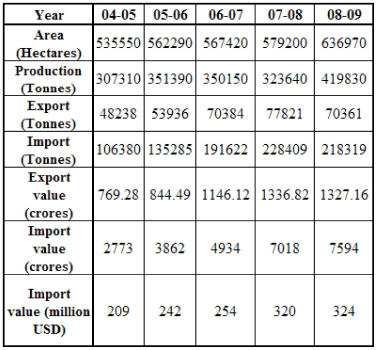Q. Approximately, what percentage of domestic consumption are imports of dry fruits during 2005-2006?

Solution:

In 2005-2006, the domestic consumption is 351390 - 53936 = 297454 tonnes
Imports in that year = 135285 The imports as a percentage of domestic consumption.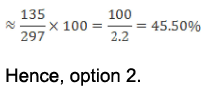QUESTION: 15

The table below shows production area, production quantity, export quantity, import quantity, export and import value of dry fruits over the period of five years in a country. The production is consumed in two ways - export and domestic consumption.

The export and import values in crores are in the local currency of the country.Q. What is the value of exports in 2008-09 in millions of US dollars, considering the same foreign exchange value as imports in that year?

Solution:

As 7594 crores in local currency correspond to 324 million USD, Each unit of local currency in 2008-2009 .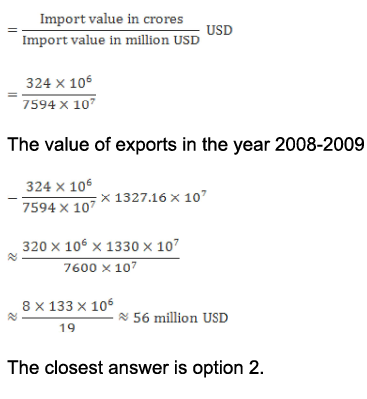Hence, option 2.

QUESTION: 16

The table below shows production area, production quantity, export quantity, import quantity, export and import value of dry fruits over the period of five years in a country. The production is consumed in two ways - export and domestic consumption.

The export and import values in crores are in the local currency of the country.Q. Which of the following statements is true?

Solution:

Consider option 1: Production per hectare in 2004-2005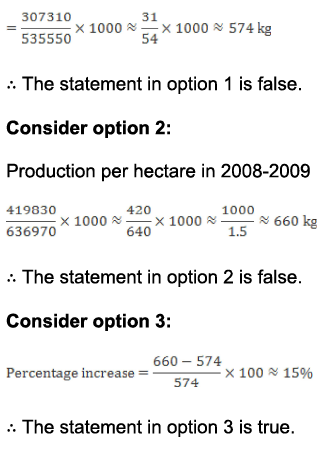Hence, option 3.

QUESTION: 17

The table below shows production area, production quantity, export quantity, import quantity, export and import value of dry fruits over the period of five years in a country. The production is consumed in two ways - export and domestic consumption.

The export and import values in crores are in the local currency of the country.Q. Which of the following statements is true?

Solution: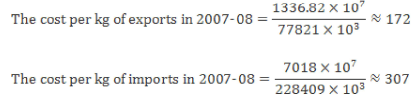The difference in costs of exports and imports = 307 - 172 = Rs. 135
Hence, option 1.

QUESTION: 18

The table below shows production area, production quantity, export quantity, import quantity, export and import value of dry fruits over the period of five years in a country.The production is consumed in two ways - export and domestic consumption.

The export and import values in crores are in the local currency of the country.Q. Which of the following statements is false?

Solution:

Consider option 1: Total number of hectares required in 2013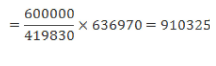Additional area needed = 910325 - 636970 = 273355 hectares or 2.73 lakh hectares.
Consider option 2: Total number of hectares required in 2013.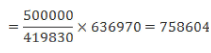Additional area needed = 758604 - 636970 = 121634 hectares or 1.22 lakh hectares.
Consider option 3: Total number of hectares required in 2013.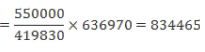Additional area needed = 834465 - 636970 = 197495 hectares or 1.98 lakh hectares.
Hence, option 3.